# Why Marginal Tax Rates Belong In Physics ClassPhoto by Kelly Sikkema on Unsplash

It’s tax season, which means most adults in the US are currently figuring out how to file their taxes, and most kids vaguely know about taxes as a thing they’ll need to do in the future. When my students feel particularly disconnected from school, they tend to ask, “When are you going to teach us something we actually need to learn, like taxes?”

As far as I’m aware, the calculation of taxes owed as a function of income is nowhere in the mandated high school curriculum. My physics students can calculate the location of an object at any future time given its history of velocities, even though most of them will never need to do this as adults. And when I realized that these physics calculations are exactly the same as marginal tax rates, I knew: taxes should be the capstone of the constant velocity motion unit in our physics classes.

Let’s start with how marginal tax rates work. The IRS sets federal tax rates, which are given here for 2021. (Note: I only ask students to look at single filers to start with.)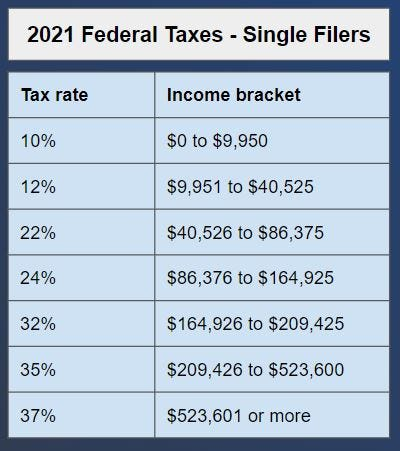IRS federal tax rates for single filers in 2021

Students’ first inclination is to look at the person’s income and take 10% if they earned \$9,950 or less, 12% if they earned between \$9,951 and \$40,525, etc. But this creates a perverse incentive. I ask students to calculate the money that would be kept after taxes for someone who made \$9,950 versus \$9,951. The person earning \$9,950 would keep \$9,950 — (10%*\$9,950) = \$8,955. The person earning \$9,951 would keep \$9,951 — (12%*\$9,951) = \$8,757. By earning one dollar more, you’d lose about \$200. This would be weird and is NOT how our system works. (For a more thorough explanation, check out Math with Bad Drawings by Ben Orlin.)

Instead, we use something called marginal tax rates. When I was about 10 years old, my father explained marginal tax rates using a bucket analogy: you think about the money you earn in a given year as if it came to you dollar by dollar. You put each dollar in the first bucket until the bucket is full. Then you begin to fill the next bucket, and so on. Each bucket gets taxed a different percentage. You take all of the percentages separately and add up the amounts to get the total you pay in taxes.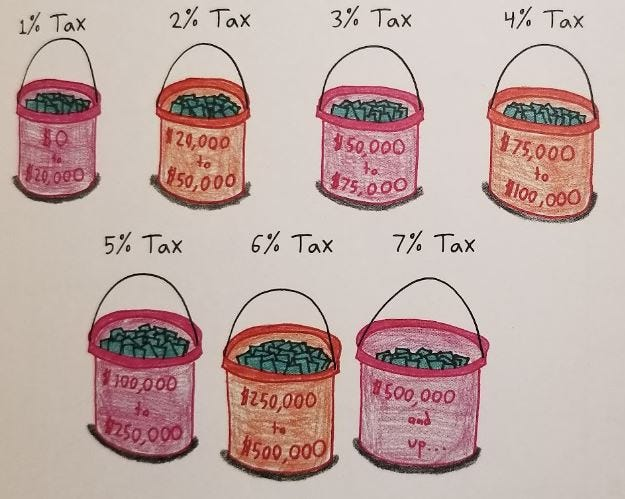Bucket analogy from Math With Bad Drawings, reproduced with author’s permission

In physics class, we study rates. A rate is a specific amount for each unit of something else. Velocity is a rate of how far you go for every unit of time that passes. We can measure velocity in meters per second (for example). Taxes are a rate of what you owe the government for every unit of money earned. These rates can change as the underlying amount changes. In physics we can study an object that moves at a certain velocity for the first few seconds and then has a different velocity for the next few seconds. With taxes, we can analyze a situation where there’s one tax rate for the first few dollars of income and then a different tax rate for subsequent dollars of income.

My students use the Desmos graphing calculator to plot tax rates as a function of income, using curly brace notation for the piecewise components. Feel free to play with the graph here. This graph of tax rates could easily be instead a graph of an object’s velocity versus time. These velocities are typical of an ant, so let’s say this graph represents the motion of an ant along the floor.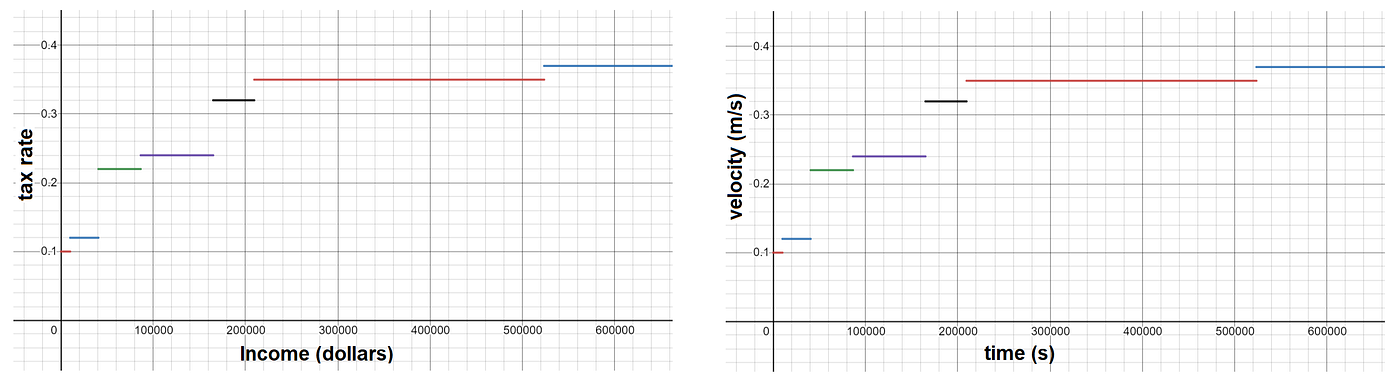Graph of actual IRS tax rates versus income, which could also be velocity versus time

Before we start discussing taxes, my physics students have already learned that they can figure out the distance an ant (for example) traveled by taking the area under the velocity-time graph: if you want to know how far something has moved, you can start with how fast it’s going (which is a rate) and multiply by the amount of time that it was moving at that rate. (Quick terminology note: since our examples here include only positive velocity in one dimension, I’m going to use “speed” and “velocity” interchangeably and the more colloquial “distance” instead of “displacement.”)

If our ant moves at 0.1 meters per second (m/s) for 5,000 seconds (which is a bit over an hour), then it travels 0.1*5,000 = 500 meters. This can be visualized as the area of the rectangle on the left. Alternatively, if the ant moves for 9,950 seconds, then it is 0.1*9,950 = 995 meters from where it began.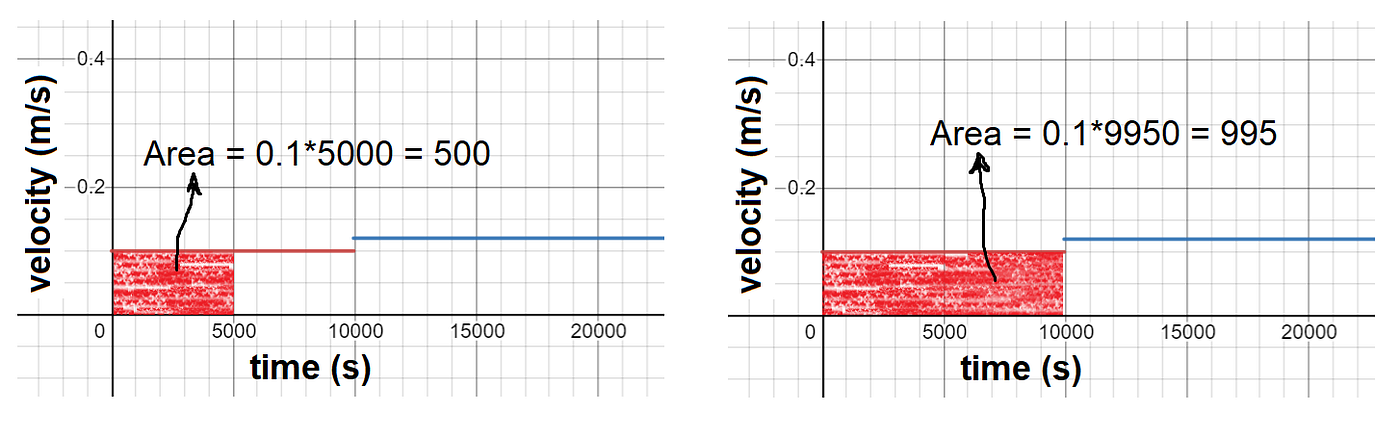Distance of an ant traveling for 5000 seconds (left) and for 9950 seconds (right)

What if, after traveling 995 m, the ant speeds up a bit, to 0.12 m/s? Well, in that case the ant’s total distance will be 995 m plus 0.12 m/s multiplied by the additional time it walks. For a concrete example, let’s say the ant walks for 20,000 seconds in total (which is about five and a half hours). To see how far it’s gone, we have to separate the journey into two parts: the first 9,950 seconds when the rate was 0.1 m/s, and the next 20,000–9,950 = 10,050 seconds when the rate was 0.12 m/s. By taking the area under the velocity-time graph, we see the ant has gone 2,201 meters in 20,000 seconds.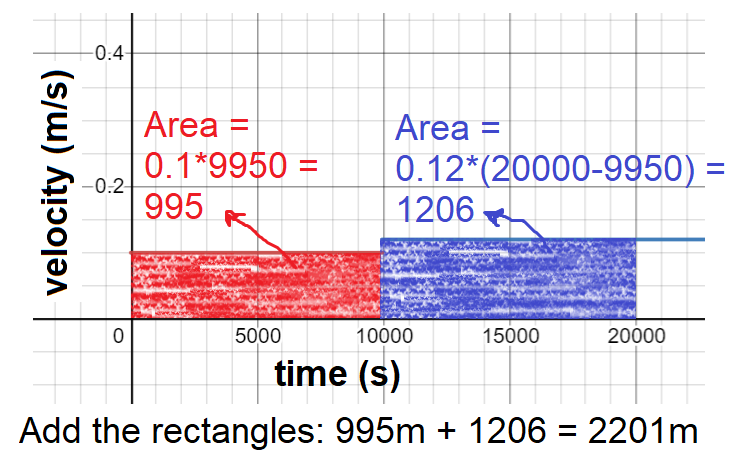Total distance after the ant increased its velocity to 0.12 m/s at the 9950-second mark

Another common physics question is: what is the ant’s average speed over the course of these 20,000 seconds? Students have learned to calculate this by taking the total distance divided by the total time: 2,201 m / 20,000 s = 0.11 m/s.

At a certain point, my students question the practicality of this lesson: most adults rarely find themselves needing to calculate total distance or average speed in this way. To the extent that we need to estimate whether a friend will make it to our house on time, we can use rough numbers: taking the area under a piecewise step function is usually unnecessary. However, this math shows up in every working adult’s life when they pay federal income taxes.

Instead of an ant traveling at 0.1 m/s for 5,000 seconds, what if we had a person who earned \$5,000 in 2021? Because the federal tax rate is 10% for single filers earning up to \$9,950, the federal taxes owed would be 0.1*5,000 = \$500. In fact, the graphs above are exactly the same if you replace the horizontal axis with “income” and the vertical axis with “tax rate.”

Let’s calculate the federal taxes owed by a first-year teacher with \$57,000 income, filing singly. As you can see from the diagram, it adds up to \$8,288.50. Each rectangle corresponds to one of the “buckets” from the bucket analogy above.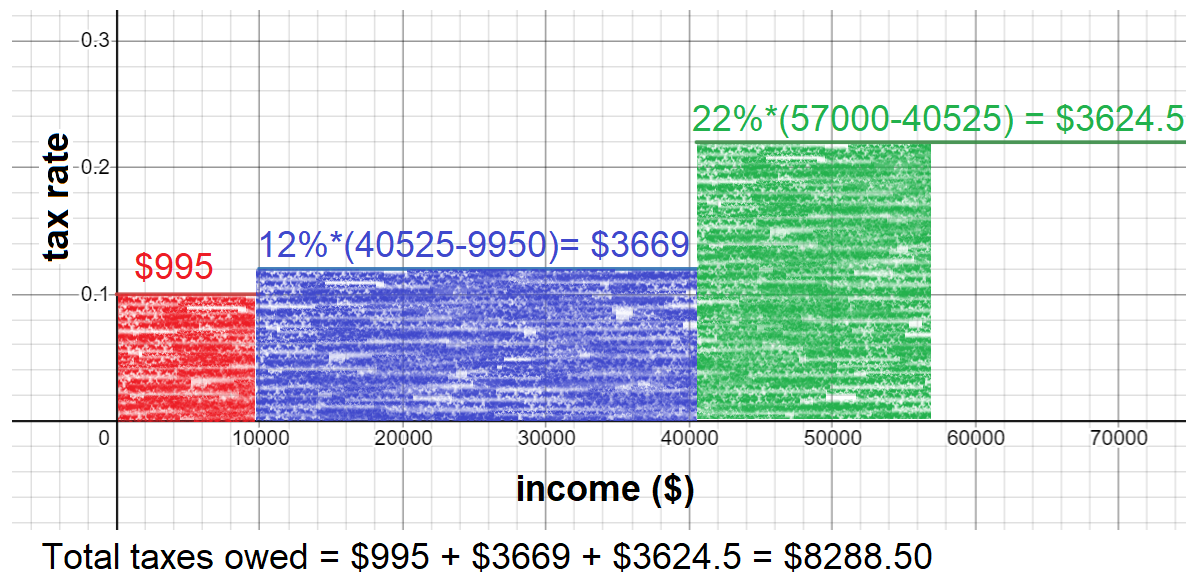Calculating federal taxes for a person with \$57000 income

This is the same process we used to calculate the ant’s distance: the sum of the rectangles’ areas determines the total federal taxes owed. And the average (or “effective”) tax rate is taxes owed divided by the total income: \$8,288.50 / \$57,000 = 14.5%.

At this point I provide students with part of the table that one can easily find by Googling “IRS tax rates.” By taking the area under the tax rates graph, they can fill in all the blanks in the third column.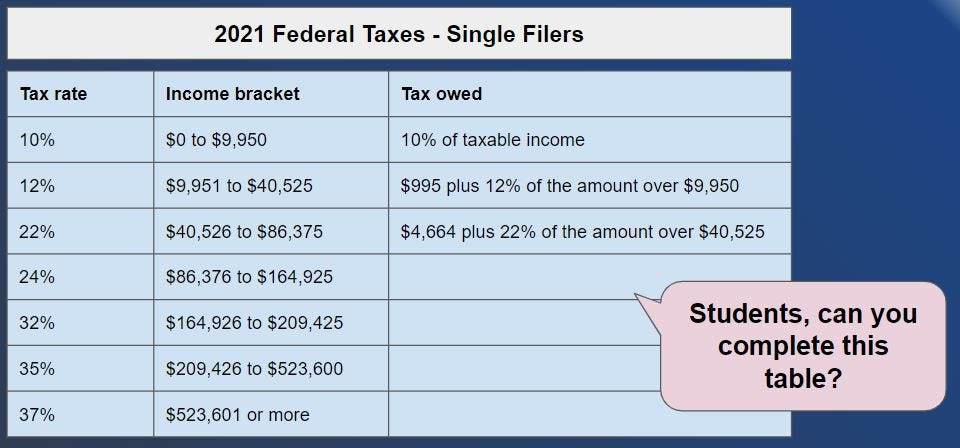Students take the area under the tax rates graph to complete column 3

Students can then generalize these calculations to graph taxes owed or money kept after taxes as a function of income. It’s a great exercise for students to generate these graphs based on the IRS tax rates, and then to interpret the graphs. For example: both graphs appear to have curvature; what does the curvature tell us about tax rates? I ask students to Google the terms “progressive tax,” “regressive tax,” and “proportional tax,” and then to explain how different marginal tax structures would change the curvature. They also opine on what tax structure they’d impose if they were in charge of the legislature. It inspires some great discussion! Some common questions they ask themselves are: (1) Why do we need to pay taxes in the first place? (2) Why are the upper and lower bounds of each tax bracket not round numbers? (3) How have these tax rates changed over time? What’s the highest they’ve ever been? Students resolve these questions through collaborative Googling and discussion.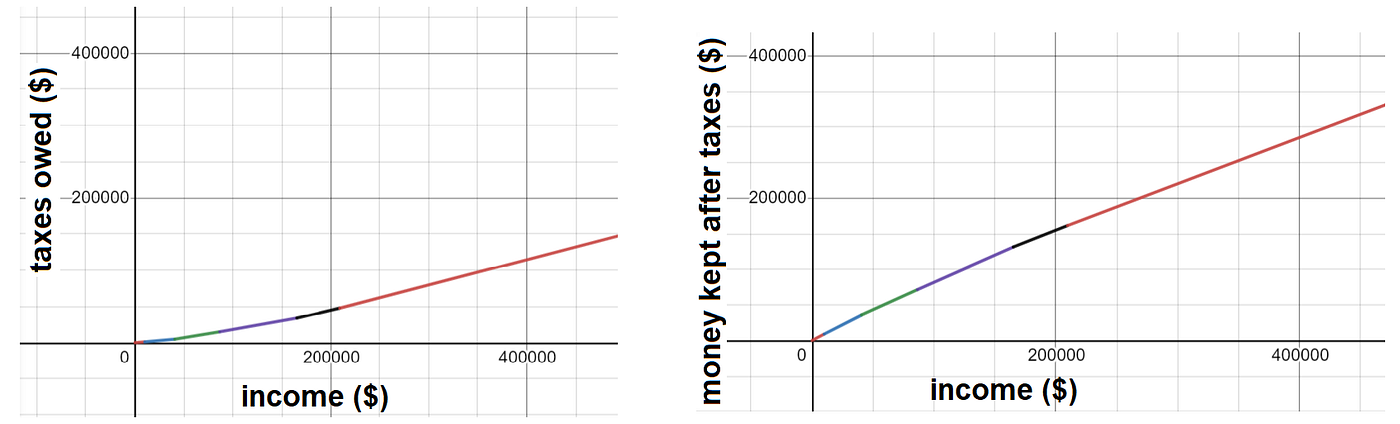Student-constructed graphs of taxes owed (left) and money kept (right) versus income

Of course this scenario is oversimplified: we’re talking about single filers and federal taxes only (no state or local). We exclude deductions, pretax spending, retirement accounts, investment-related taxes, etc. As I enumerate all the pieces we don’t address in my class, students begin to appreciate how complicated taxes are. They can see why most people use online programs or pay someone else to calculate their taxes for them. My students, too, will probably end up using off-the-shelf software when they start paying taxes. But at least they’ll have a deeper sense of what the software is doing, and hopefully they will be empowered to ask questions when they see outputs that don’t make sense.

I’m now ready and eager for the common physics student question: “When in real life will we ever need to know how to calculate the total distance of a person who traveled at 2 m/s for 5 seconds, then 4 m/s for 3 seconds, then 6 m/s for 2 seconds?”

The answer is: you’ll need this every year when you do your taxes.

(Also: I’m happy to share the slides and worksheets I created for this lesson. Please reach out if you’re interested.)

--

--